Homework Help Question & Answers

# Guestion 4 (1 point) A 34.1 gram piece of lead at 96.9。C is placed in a...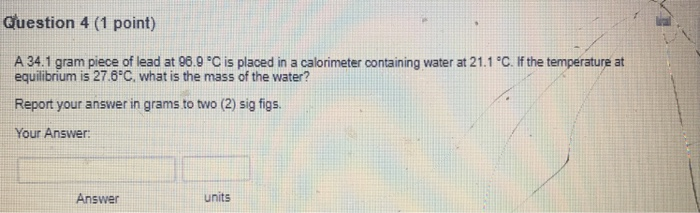Guestion 4 (1 point) A 34.1 gram piece of lead at 96.9。C is placed in a calorimeter oontaining water at 21.1°C. If the temperature at equilibrium is 27.8C, what is the mass of the water? Report your answer in grams to two (2) sig figs Your Answer: Answer units

#### Homework Answers

Answer #1

Heat lost by lead= heat gained by water

=> mlead x clead x (Tf -Ti) = mwater x Cwater x ( Tf - Ti)

=> 34.1 g x 0.160 J/g0C x( 96.9 -27.6)0C = mwater x 4.184 J/g0C x ( 27.6 - 21.1)0C

=> 378.1008 J = mwater x 27.196 J/g

=> mwater =( 378.1008 /27.196) g

=> mwater = 13.9 g = 14 g ( 2 sig. figures)

Know the answer?
Your Answer:

#### Post as a guest

Your Name:

What's your source?

#### Earn Coin

Coins can be redeemed for fabulous gifts.

Not the answer you're looking for? Ask your own homework help question. Our experts will answer your question WITHIN MINUTES for Free.
Similar Homework Help Questions
• ### Question 21 (1 point) ✓ Saved A 40.1 gram piece of lead at 97.7 °C is...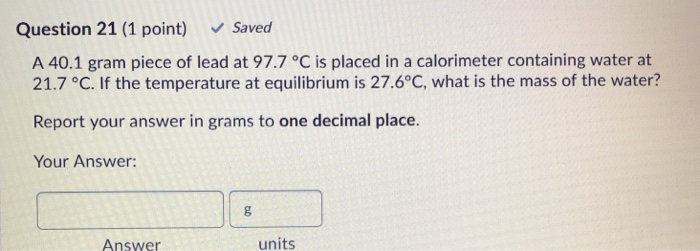Question 21 (1 point) ✓ Saved A 40.1 gram piece of lead at 97.7 °C is placed in a calorimeter containing water at 21.7 °C. If the temperature at equilibrium is 27.6°C, what is the mass of the water? Report your answer in grams to one decimal place. Your Answer: 8 Answer units

• ### Question 4 4 pts A piece of metal with a specific heat of 1.29 J/gºC is...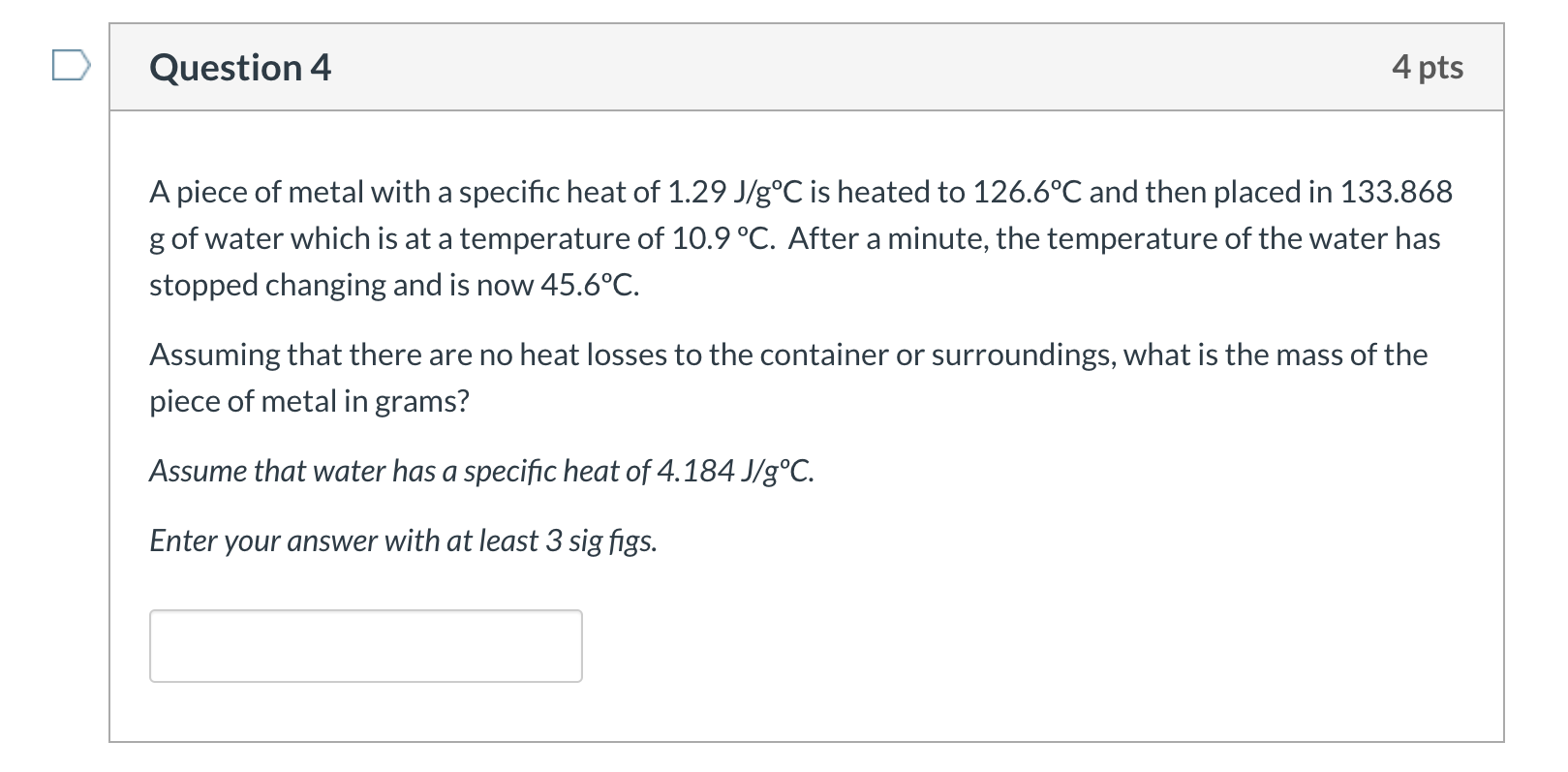Question 4 4 pts A piece of metal with a specific heat of 1.29 J/gºC is heated to 126.6°C and then placed in 133.868 g of water which is at a temperature of 10.9 °C. After a minute, the temperature of the water has stopped changing and is now 45.6°C. Assuming that there are no heat losses to the container or surroundings, what is the mass of the piece of metal in grams? Assume that water has a specific heat...

• ### A piece of metal with a specific heat of 1.29 J/g°C is heated to 126.6°C and...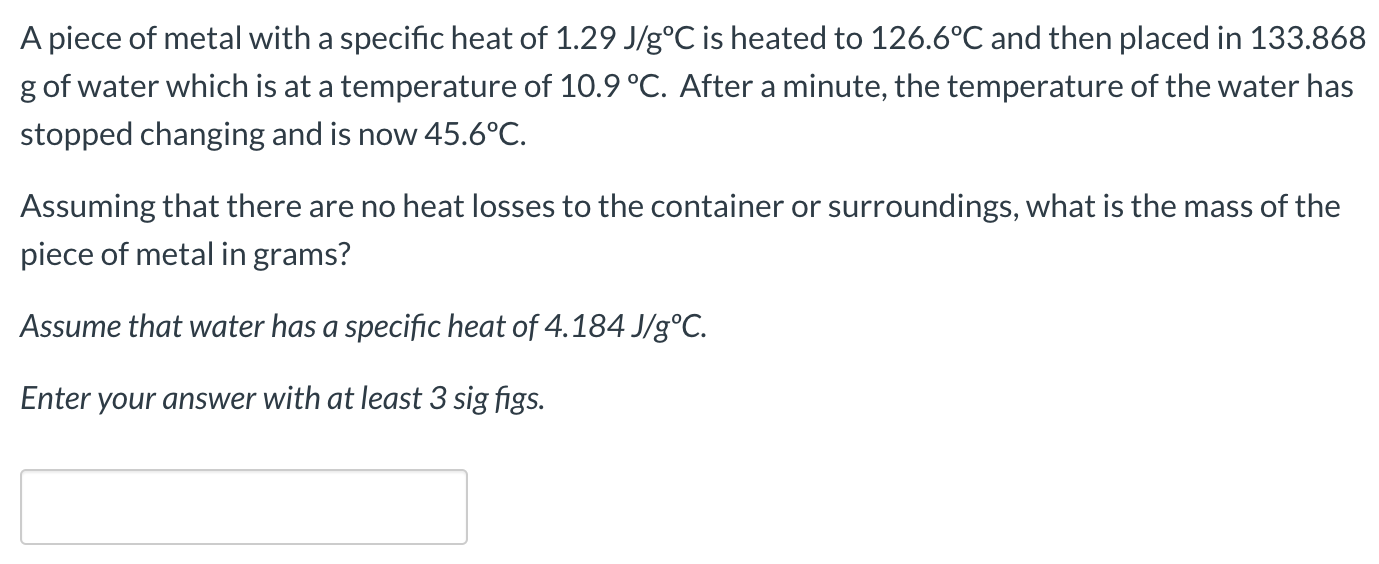A piece of metal with a specific heat of 1.29 J/g°C is heated to 126.6°C and then placed in 133.868 g of water which is at a temperature of 10.9 °C. After a minute, the temperature of the water has stopped changing and is now 45.6°C. Assuming that there are no heat losses to the container or surroundings, what is the mass of the piece of metal in grams? Assume that water has a specific heat of 4.184 J/g°C. Enter...

• ### 4. A 0.500 kg piece of copper at an initial temperature of 20.0°C is placed in...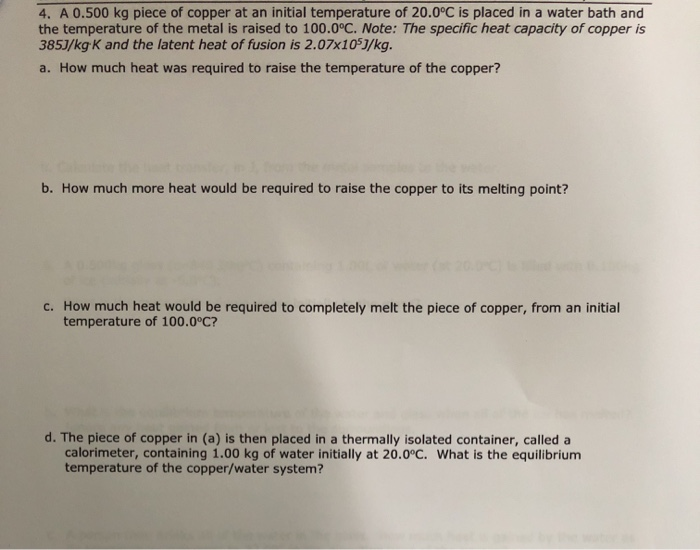4. A 0.500 kg piece of copper at an initial temperature of 20.0°C is placed in a water bath and the temperature of the metal is raised to 100.0°C. Note: The specific heat capacity of copper is 385J/kg K and the latent heat of fusion is 2.07x1057/kg. a. How much heat was required to raise the temperature of the copper? b. How much more heat would be required to raise the copper to its melting point? C. How much heat...

• ### 5) A 3.50 kg piece of lead and a 1.5 kg piece of aluminum are placed...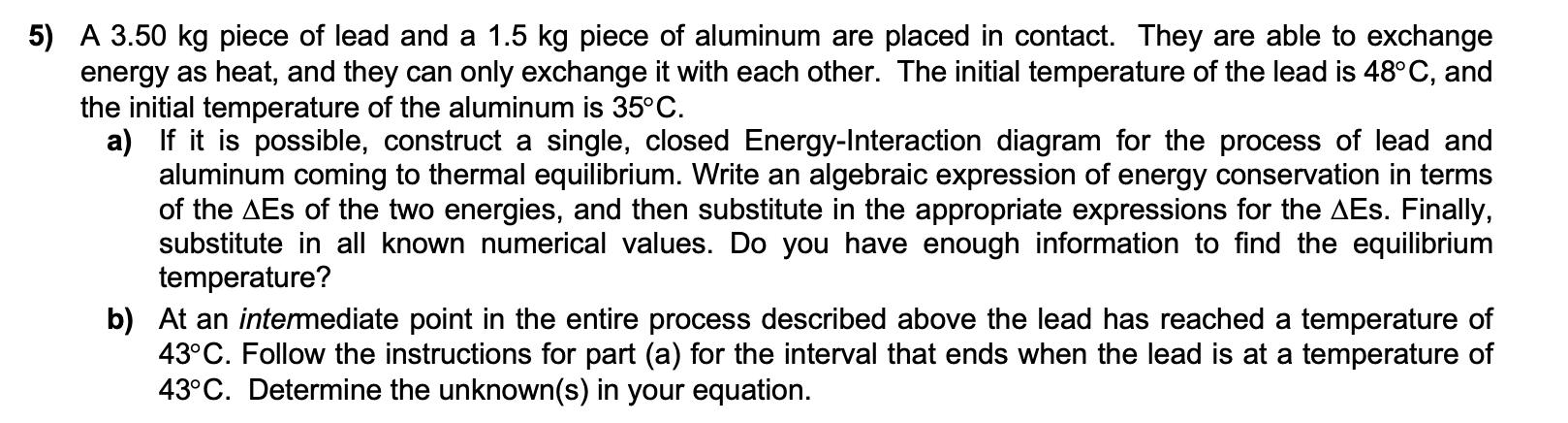5) A 3.50 kg piece of lead and a 1.5 kg piece of aluminum are placed in contact. They are able to exchange energy as heat, and they can only exchange it with each other. The initial temperature of the lead is 48°C, and the initial temperature of the aluminum is 35°C. a) If it is possible, construct a single, closed Energy-Interaction diagram for the process of lead and aluminum coming to thermal equilibrium. Write an algebraic expression of energy...

• ### A piece of solid lead weighing 34.2 g at a temperature of 315 °C is placed...

A piece of solid lead weighing 34.2 g at a temperature of 315 °C is placed in 342 g of liquid lead at a temperature of 376 °C. After a while, the solid melts and a completely liquid sample remains. Calculate the temperature after thermal equilibrium is reached, assuming no heat loss to the surroundings. The enthalpy of fusion of solid lead is ΔHfus = 4.77 kJ/mol at its melting point of 328 °C, and the molar heat capacities for...

• ### correct sig fig number please! Question 49 (1 point) A student runs the reaction below starting...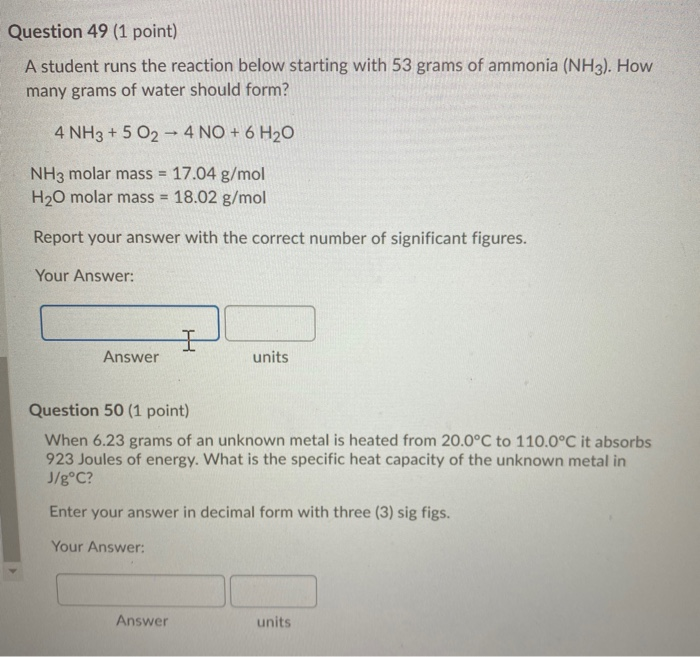correct sig fig number please! Question 49 (1 point) A student runs the reaction below starting with 53 grams of ammonia (NH3). How many grams of water should form? 4 NH3 + 5 O2 - 4 NO + 6 H20 NH3 molar mass = 17.04 g/mol H20 molar mass = 18.02 g/mol Report your answer with the correct number of significant figures. Your Answer: I Answer units Question 50 (1 point) When 6.23 grams of an unknown metal is heated...

• ### Review Part A A 215 g lead ball at a temperature of 81.9 °C is placed in a light calorimeter containing 154 g of water at 22.3 °C. Find the equilibrium temperature of the system. Express your answer...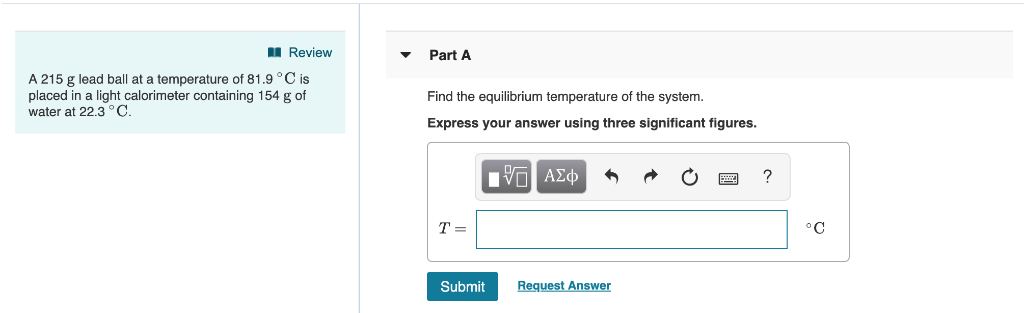Review Part A A 215 g lead ball at a temperature of 81.9 °C is placed in a light calorimeter containing 154 g of water at 22.3 °C. Find the equilibrium temperature of the system. Express your answer using three significant figures. o C Submit Review Part A A 215 g lead ball at a temperature of 81.9 °C is placed in a light calorimeter containing 154 g of water at 22.3 °C. Find the equilibrium temperature of the system....

• ### 4. A 0.500 kg piece of copper at an initial temperature of 20.0°C is placed in...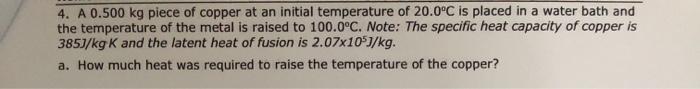4. A 0.500 kg piece of copper at an initial temperature of 20.0°C is placed in a water bath and the temperature of the metal is raised to 100.0°C. Note: The specific heat capacity of copper is 385J/kg K and the latent heat of fusion is 2.07x105J/kg. a. How much heat was required to raise the temperature of the copper? e. An identical piece of heated copper (at 100.0°C) is placed in a calorimeter containing 0.500 kg of an unknown...

• ### What is the final temperature, (in oC), after a 26.5 gram piece of ice at 0oC...

What is the final temperature, (in oC), after a 26.5 gram piece of ice at 0oC is placed into a styrofoam cup with 117.0 grams of water initially at 76.5oC? Assume no loss or gain of heat from the surroundings. Enter your answer without units. heat of fusion of water is 333 J/g. The specific heat of H2O(l) is approximately constant at 4.184 J/gK.

Free Homework App

Scan Your Homework
to Get Instant Free Answers
Need Online Homework Help?

Get Answers For Free
Most questions answered within 3 hours.